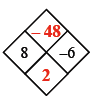### Home > CC3MN > Chapter 3 Unit 3 > Lesson CC3: 3.2.4 > Problem3-102

3-102.

Copy and complete each of the Diamond Problems below. The pattern used in the Diamond Problems is shown at right.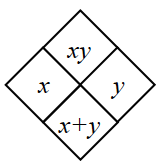1.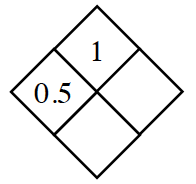1. Divide $1$ by $0.5$ to find $y$.

Add $x$ and $y$ together to get the bottom of the diamond.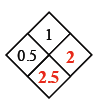1.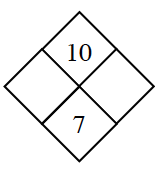What are the factors of $10$?

Which of those add to $7$?

1.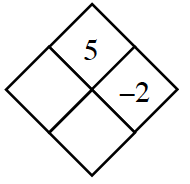Follow the steps in part (a).

1.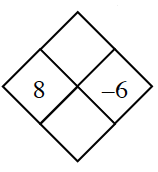Use the pattern above to find $xy$ and $x+y$.